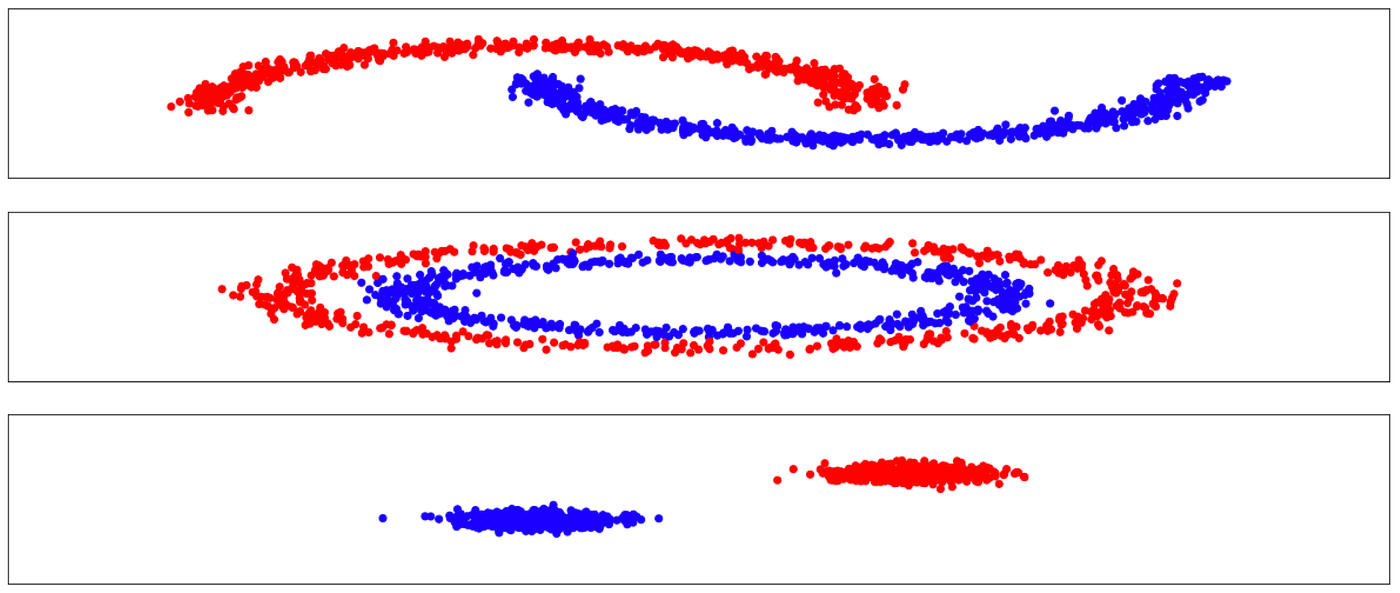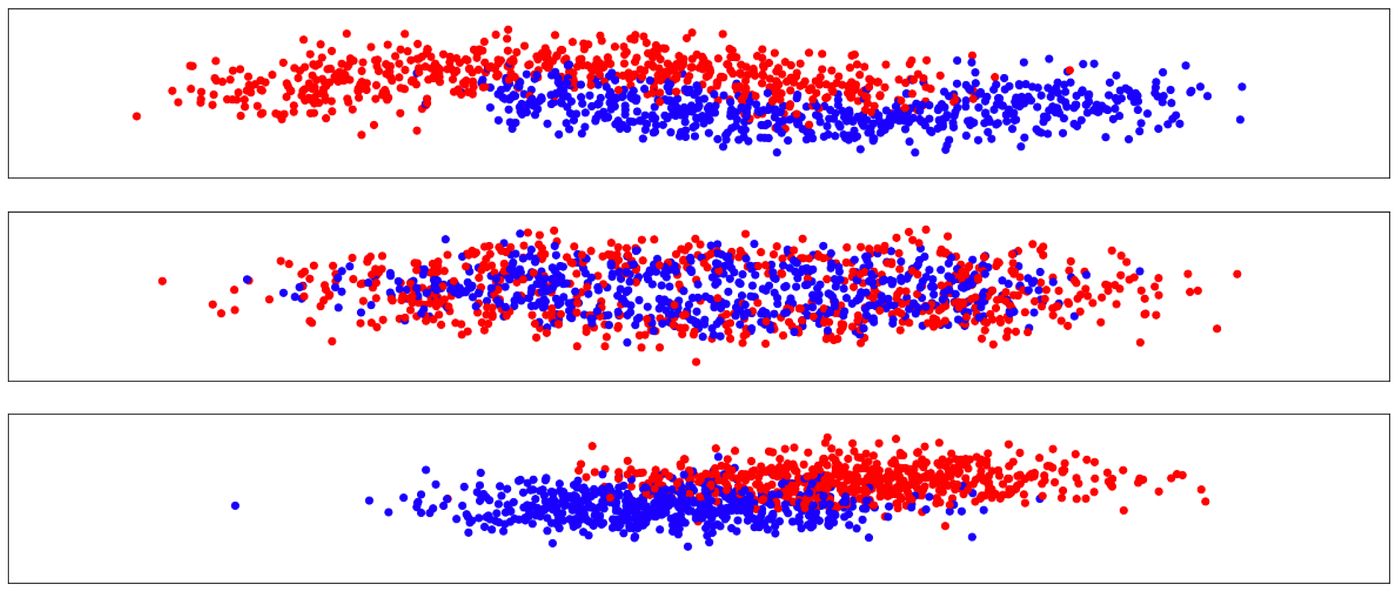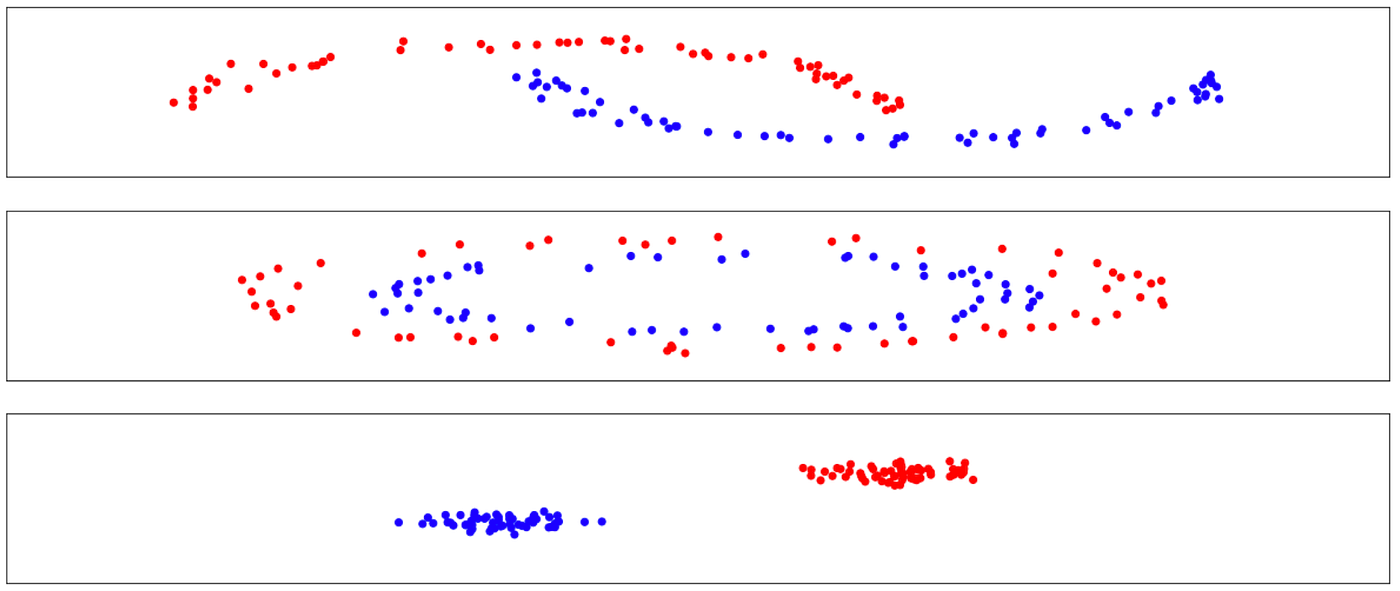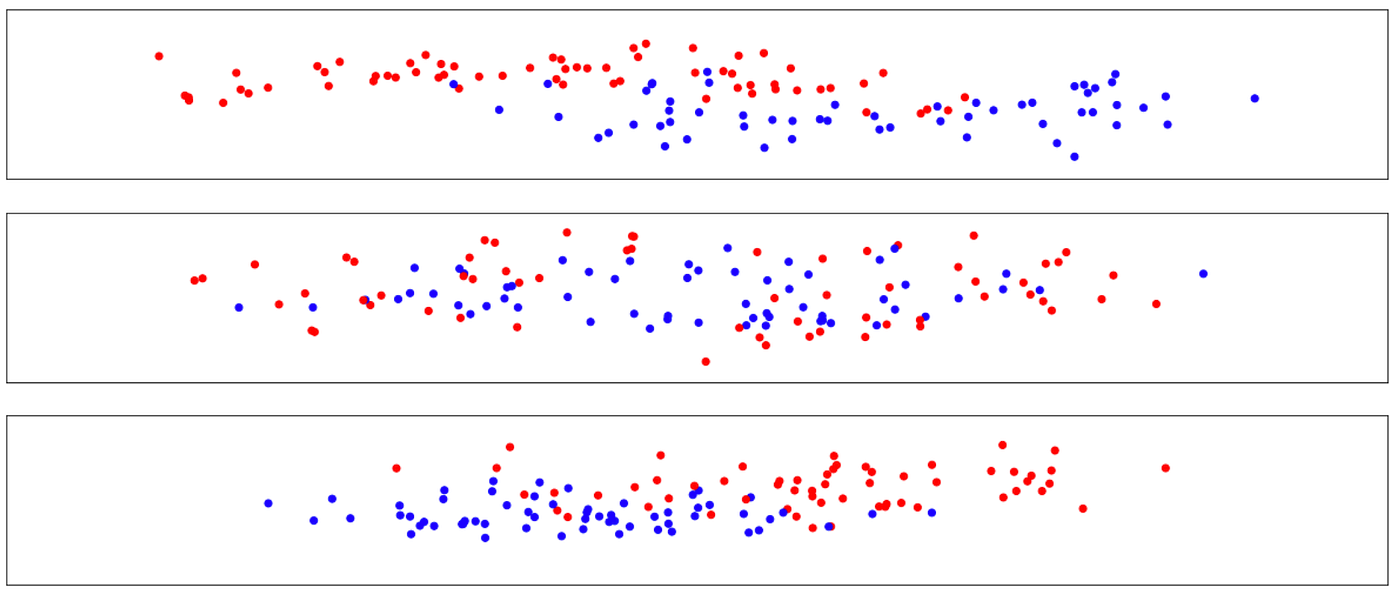# Classification with OpenCV3 C++ (1/2)

## Installing OpenCV3

`brew install opencv3 --c++11 --with-python3 --with-contrib`

## Data

`from sklearn.datasets import make_moons, make_circles, make_blobs`large samples with low variance (top: data set 1, middle: data set 2 and bottom: data set 3)large samples with high variance (top: data set 1, middle: data set 2 and bottom: data set 3)small samples with low variance (top: data set 1, middle: data set 2 and bottom: data set 3)small samples with high variance (top: data set 1, middle: data set 2 and bottom: data set 3)

## OpenCV containers

`auto array = cv::Mat::ones(1, 20, CV_32F);`
`auto zer = cv::Mat::zeros(1, 20, CV_32F); // zero matrixauto id = cv::Mat::eye(20, 20, CV_32F); // identity matrix`
`CV_<bit_depth><type>`
`auto X = cv::Mat::zeros(400,2,CV_32F); // dummy features matrixauto Y= cv::Mat::zeros(400,1,CV_32U); // dummy label matrixcv::Ptr<cv::ml::TrainData> data=cv::ml::TrainData::create(X,0,Y);`
`data->setTrainTestSplitRatio(0.2,true);`

## Binary classification

`cv::ml::Ptr<StatModel> model = cv::ml::StatModel::create();`
`model->train(data);`
`model->isTrained();`
`model->calcError(data, true, y);`
`model->predict(x_test);`
`kNearest->setDefaultK(5);kNearest->setIsClassifier(true);`
• setDefaultK: sets the number of nearest neighbours.
• setIsClassifier: if false, the algorithm fitted is a regression (i.e. continuous output) if true it is a classifier.
`decisionTree->setMaxDepth(3000);decisionTree->setMinSampleCount(1);decisionTree->setUse1SERule(false);decisionTree->setUseSurrogates(false);decisionTree->setPriors(cv::Mat());decisionTree->setCVFolds(1);`
• setMaxDepth: sets the maximum depth of the tree.
• setMinSampleCount: sets the minimum number of observation in each leaf.
• setUse1SERule: is true algorithm performs a more aggressive pruning of the tree which reduces its variance.
• setUseSurrogates: only useful if there are missing input values.
• setPriors: skews penalty for miss-classification for certain classes.
• setCVFolds: sets the cross validation procedure order for pruning the tree.
`randomForest->setMaxCategories(2);randomForest->setMaxDepth(3000);randomForest->setMinSampleCount(1);randomForest->setTruncatePrunedTree(false);randomForest->setUse1SERule(false);randomForest->setUseSurrogates(false);randomForest->setPriors(cv::Mat());randomForest->setTermCriteria(criterRamdomF);randomForest->setCVFolds(1);`
• setTermCriteria: sets the convergence criteria by choosing when the algorithm stops (either when a given precision is reached CV_TERMCRIT_EPS, or when a number of iteration is reached CV_TERMCRIT_ITER). Then the value of each criteria must be set.
`auto criterRamdomF = cv::TermCriteria();criterRamdomF.type = CV_TERMCRIT_EPS + CV_TERMCRIT_ITER;criterRamdomF.epsilon = 1e-8;criterRamdomF.maxCount = 5000;`
`boost->setBoostType(cv::ml::Boost::DISCRETE);boost->setWeakCount(100);boost->setMaxDepth(2000);boost->setUseSurrogates(false);boost->setPriors(cv::Mat());`
• setBoostType: selects the type of boosting algorithm used (options are AdaBoost or Logit).
• setWeakCount: sets the number of weak classifiers used.
`linearSvm->setC(100);linearSvm->setKernel(linearSvm->LINEAR);;linearSvm->setTermCriteria(criterSvm);linearSvm->setType(linearSvm->C_SVC);`
• setKernel: sets the kernel function.
• setC: sets the regularization hyperparameter.
`rbfSvm->setC(1000);rbfSvm->setTermCriteria(criterRbf);rbfSvm->setCoef0(0.3);rbfSvm->setKernel(rbfSvm->RBF);rbfSvm->setGamma(0.9);rbfSvm->setType(rbfSvm->C_SVC);`
• setGamma: hyperparameter of the sigmoid kernel function.
• setCoef0: hyperparameter of the sigmoid kernel function.
`sigmoidSvm->setC(1000);sigmoidSvm->setTermCriteria(criterSigmoid);sigmoidSvm->setCoef0(0.3);sigmoidSvm->setGamma(0.9);sigmoidSvm->setKernel(sigmoidSvm->SIGMOID);sigmoidSvm->setType(sigmoidSvm->C_SVC);`